<< Back

# A Comparison of Put-Call Ratios

Frank Ferstler, Nicholas Pezolano
2021-09-28

The Put-Call ratio is often the most commonly used Options-Implied Indicator due to it's simplicity in calculation. However there exists several variations in methodology to calculate it. In this blogpost we give an overview of these different methods and their relevance. The Put-Call ratio can be calculated using volume or open interest and it can be filtered by moneyness (ATM, ITM or OTM).

### Calculations

The most common variation uses the Volume of Puts to Volume of Calls. A similar ratio is also calculated using the Options Open Interest. Options Volume captures the number of contracts that exchange hands (buy or sell) whereas the open interest captures the demand for the contracts.

$$P/C~Ratio_{Volume}~=~P_{Volume} / C_{Volume}$$ $$P/C~Ratio_{OI}~=~P_{OI} / C_{OI}$$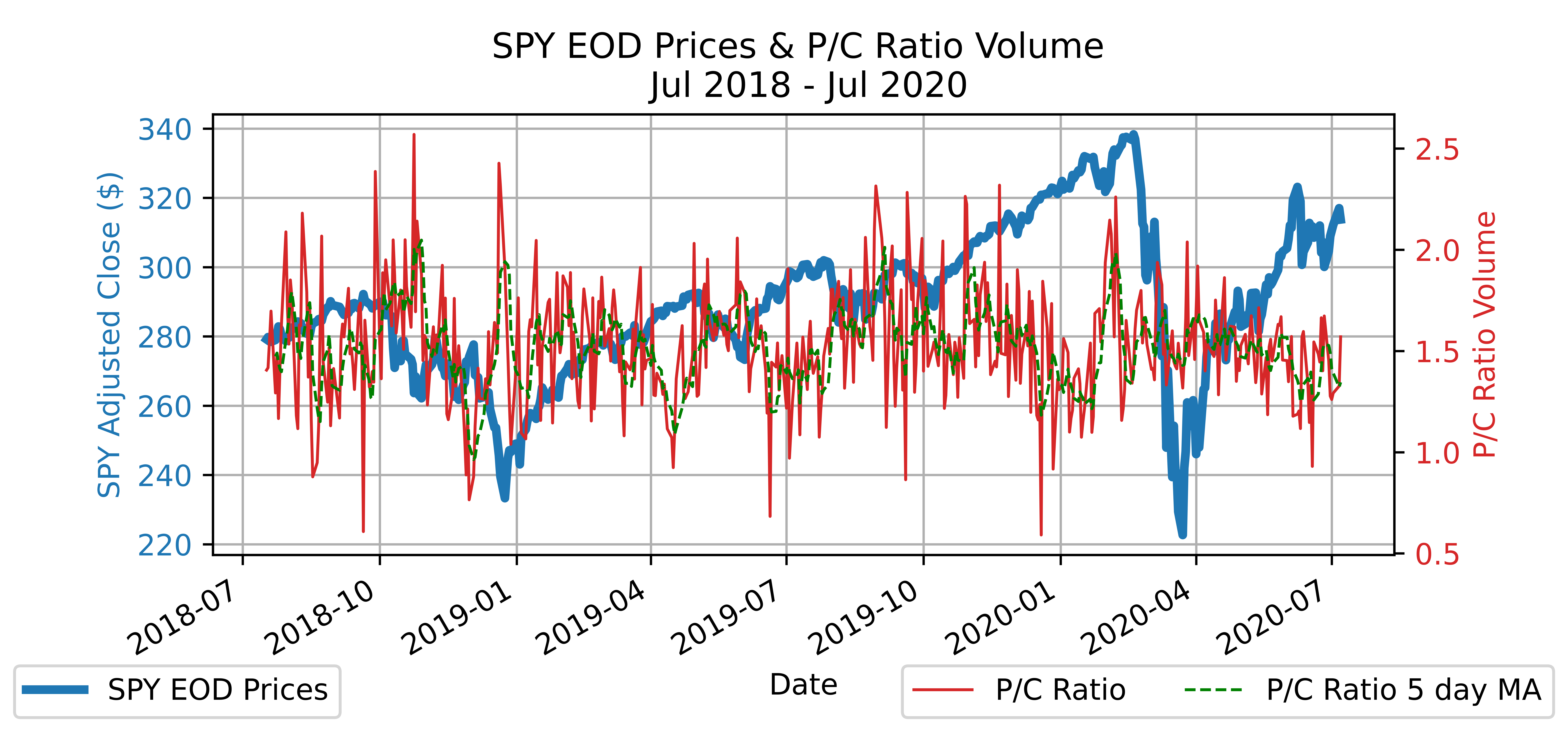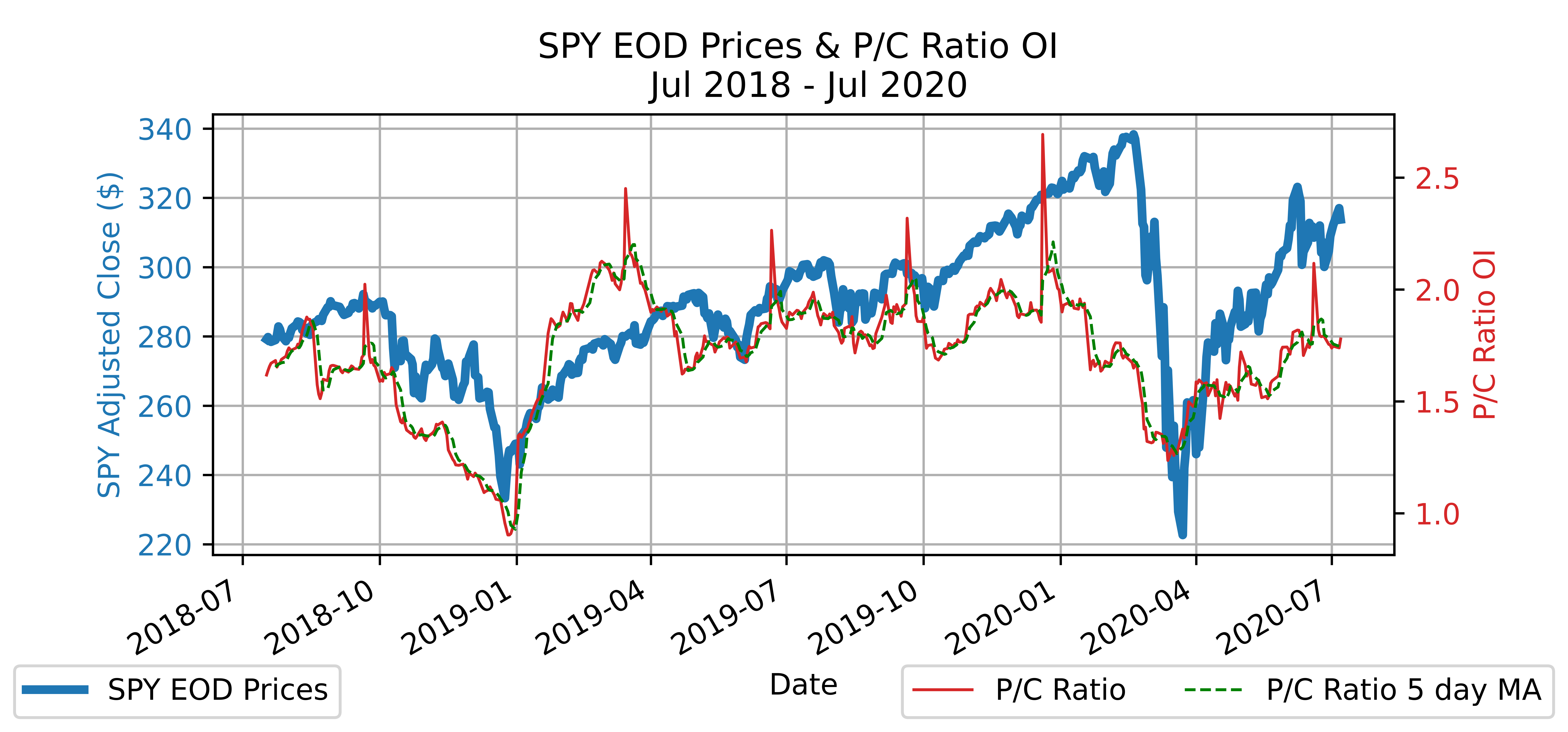Another variation compares Puts to the sum of Puts and Calls (total options). This has the convenience of being more mathematically friendly with a scale between 0 and 1. This has nice practicalities such as being stationary which can be directly used in time series analyses without the need of further transformation.

$$Puts~Percentage_{Volume}~=~P_{Volume} / (C_{Volume}~+~P_{Volume})$$ $$Puts~Percentage_{OI}~=~P_{OI} / (C_{OI}~+~P_{OI})$$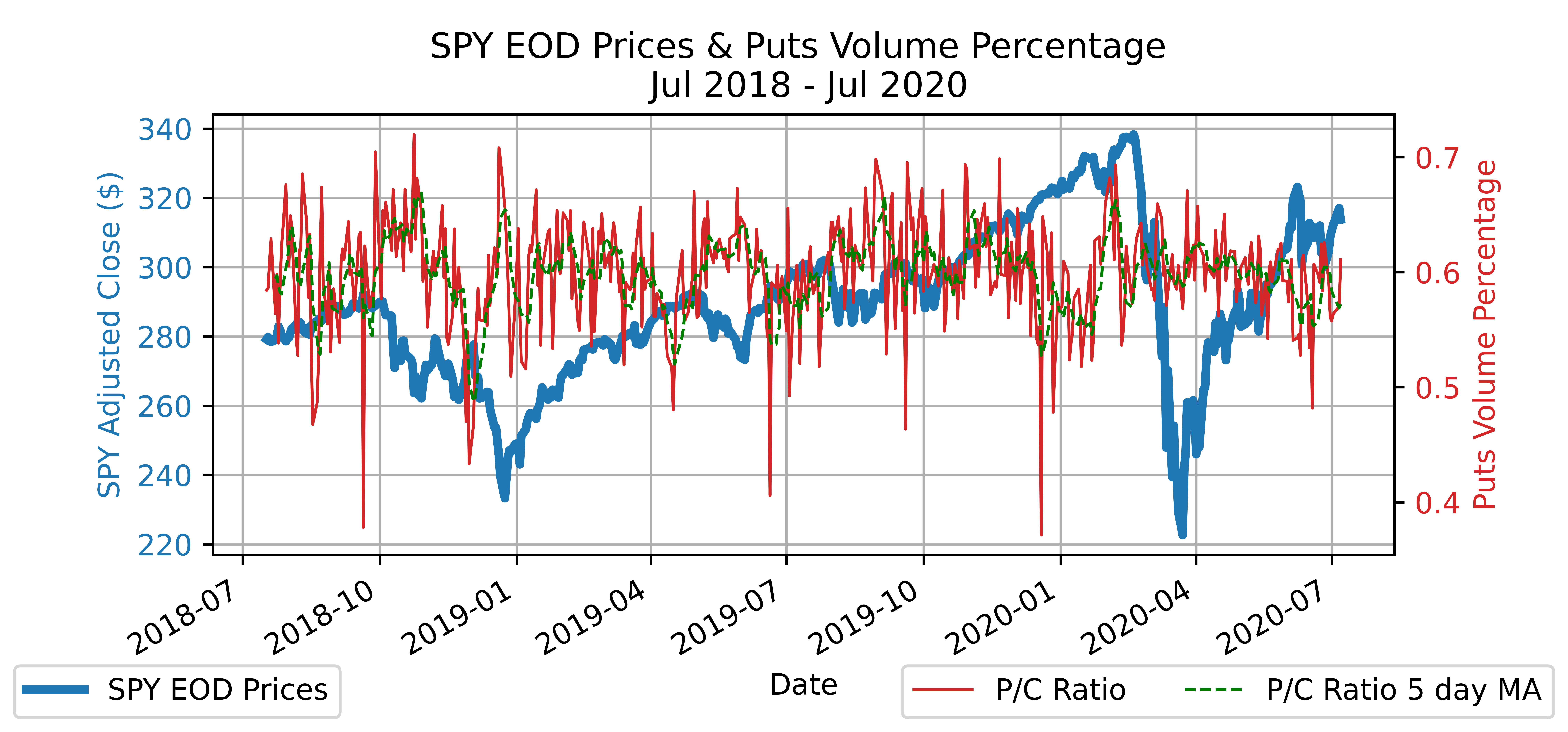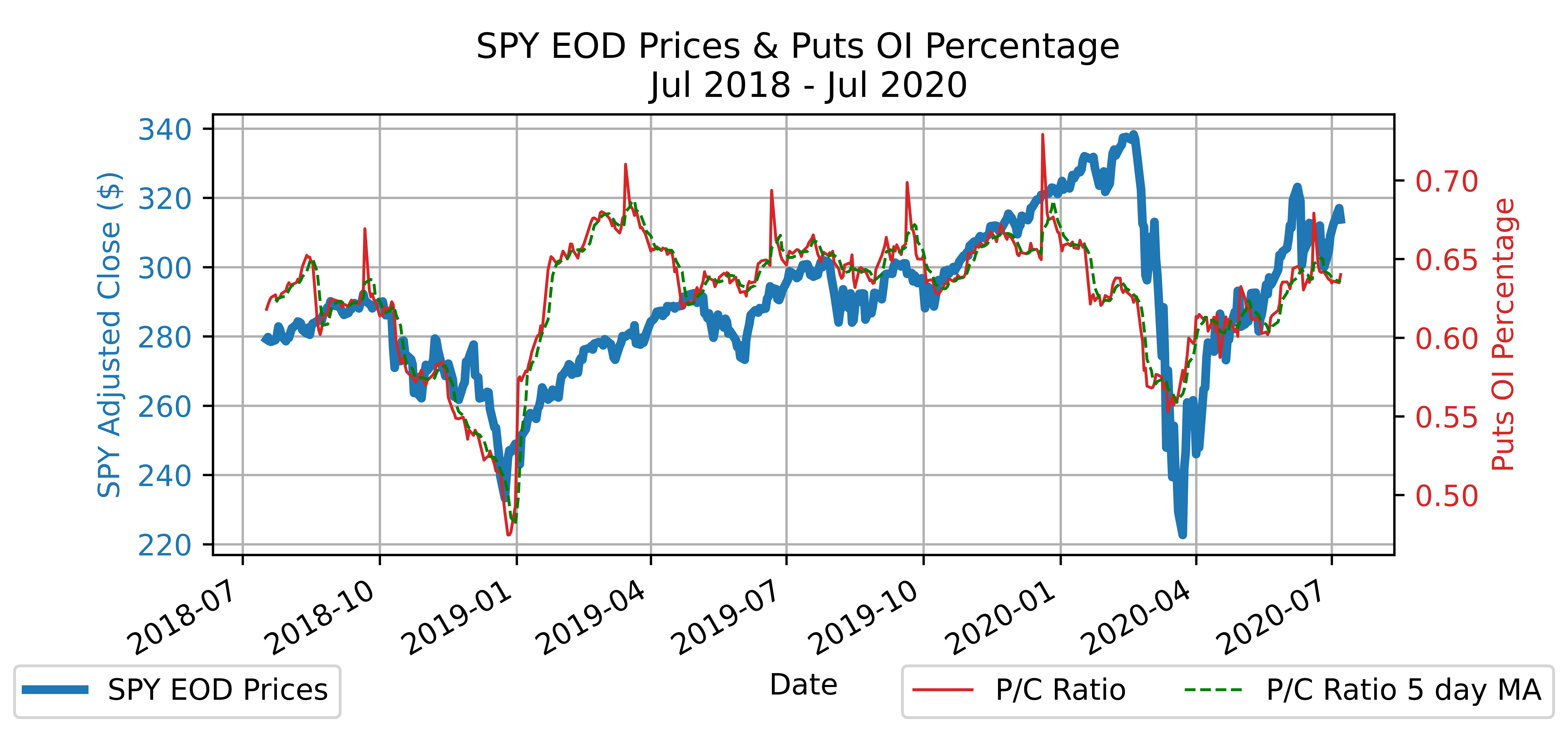Further variants of the Put-Call ratio account for the moneyness of the options. Out of the money (OTM) options can depict the market's expectation on the future price of the underlying asset. These ratios can also be analyzed as a surface which we won't get into in this post.

$$P/C~OTM~Ratio~=~P_{OTM} / C_{OTM}$$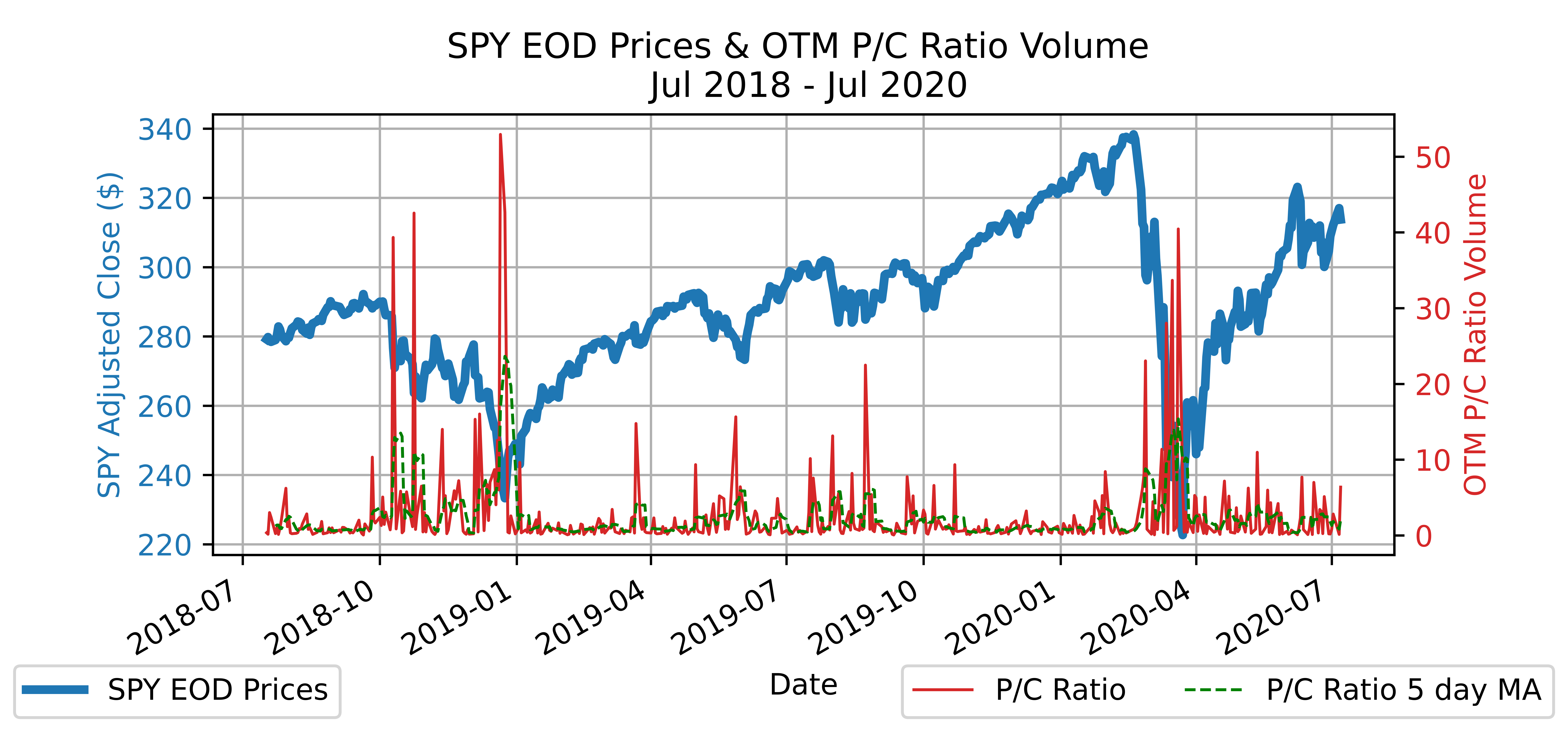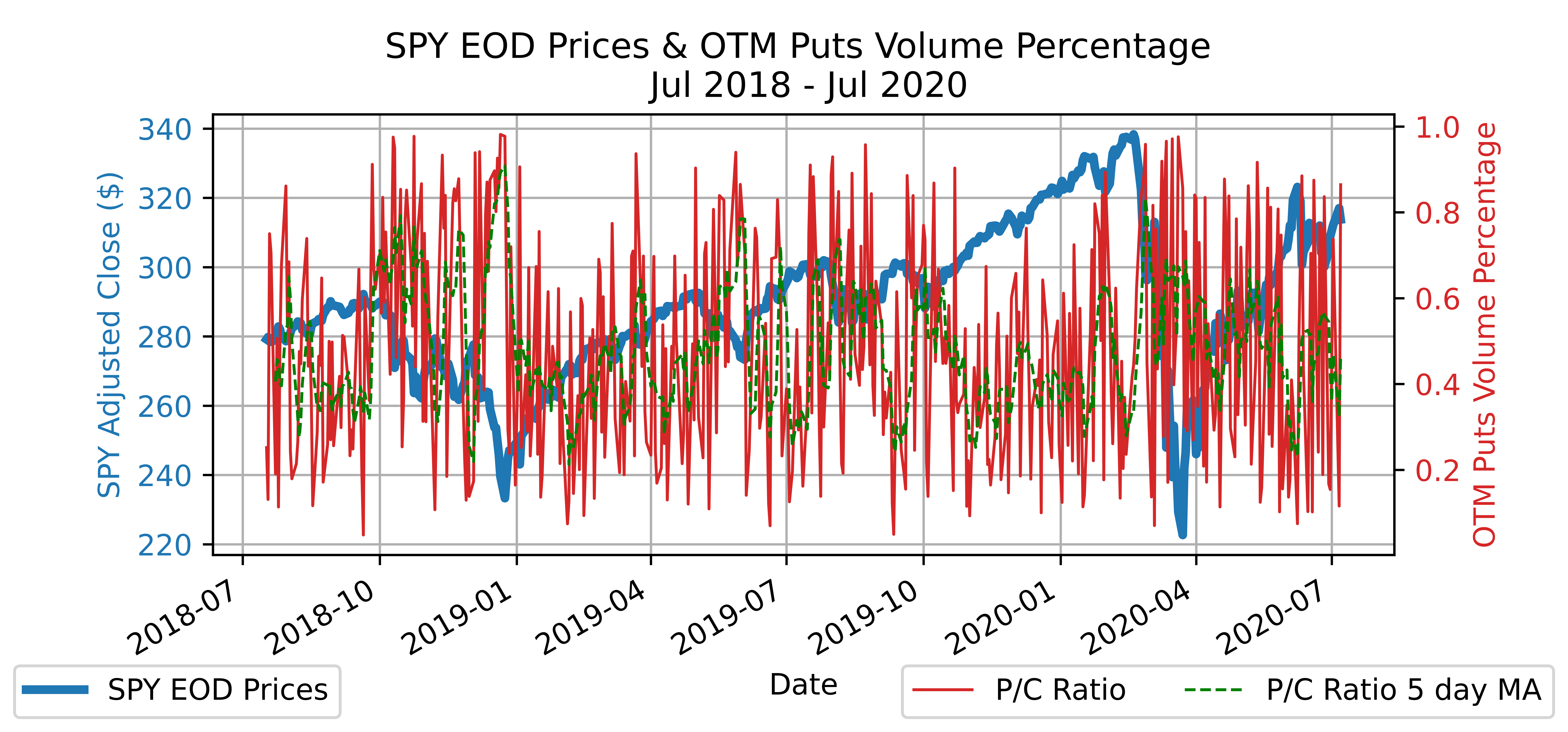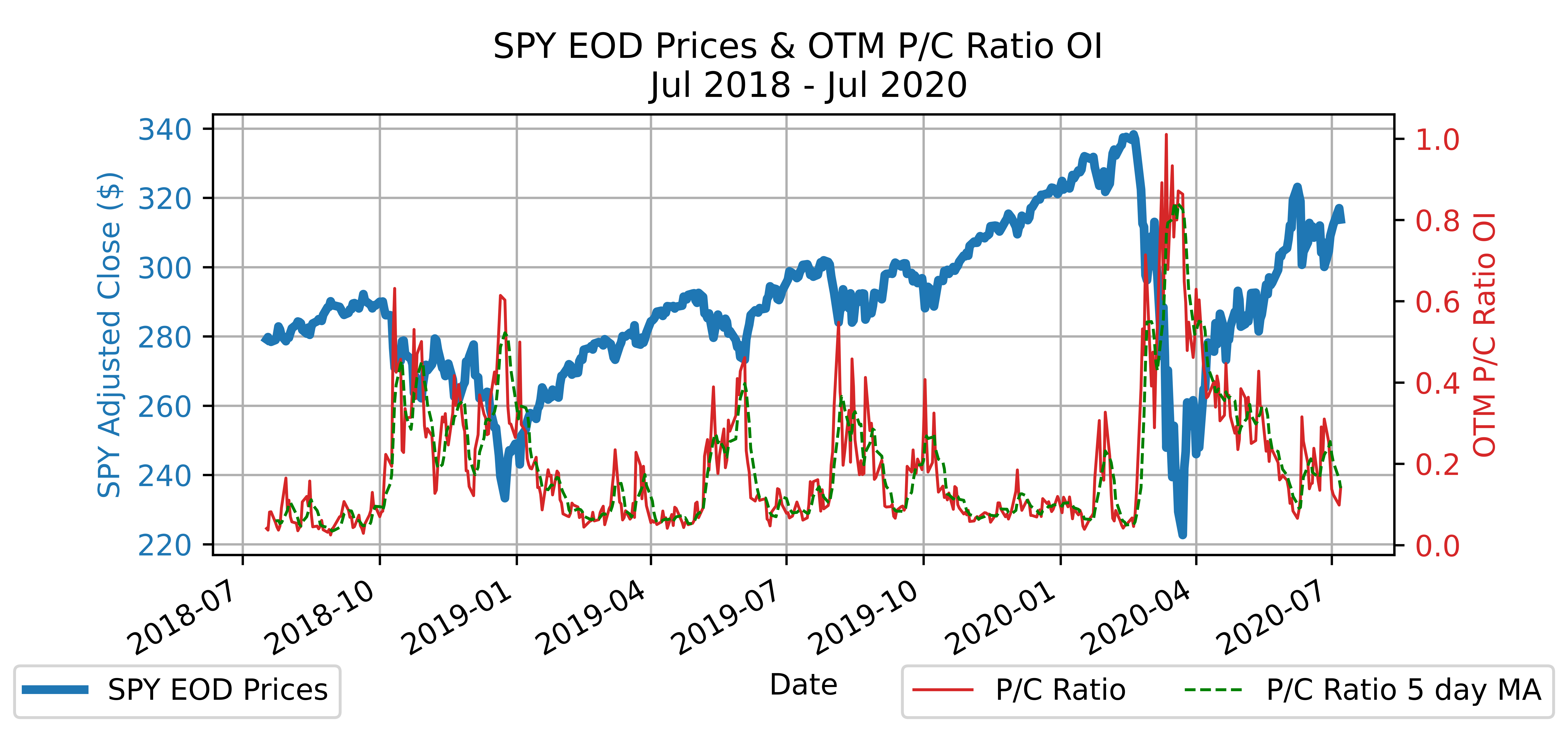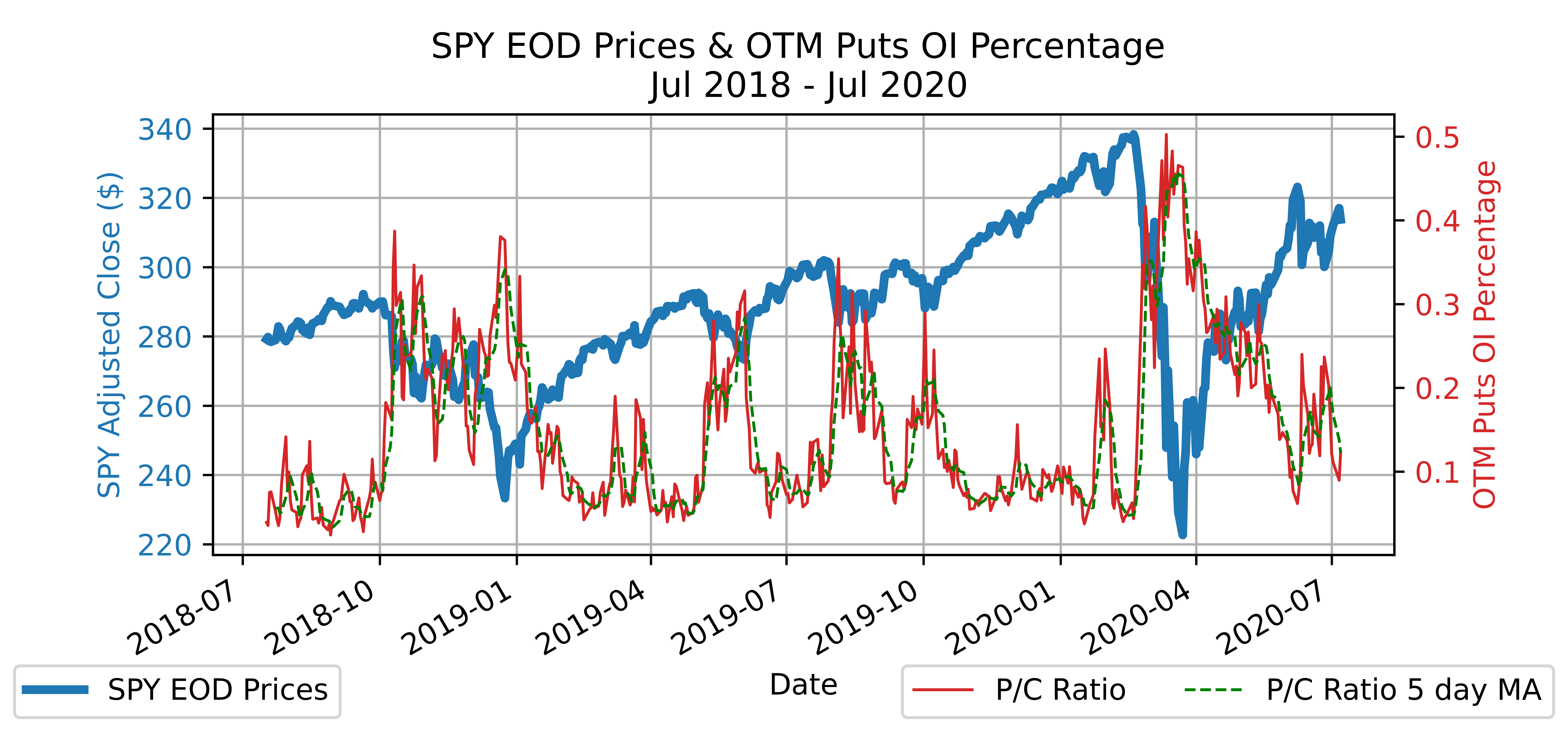### Significance

At quick glance through the different variations, one common theme is that there appears to be a relationship between the change in price and change in metric. To test the significance for each of the variants, a regression is ran following the below formulation which will aim to capture the next day's change in price.

$$\Delta SPY_{t+1}~=~\alpha~+~\beta_{1} \Delta SPY_{t}~+~\beta_{2} \Delta P/C~Ratio_{t}~+~\beta_{3} \rho_{t}~+~\beta_{4}( \Delta P/C~Ratio_{t}~\times~\rho_{t})~+~\epsilon_{t}$$
Coef Std Err Z-score
Alpha (α) 0.16 0.17 0.94
ΔSPY -0.22 0.10 -2.15
ΔP/C Ratio 22.78 18.55 1.23
Rho (ρ) -0.85 0.81 -1.05
ΔP/C Ratio * ρ 429.01 106.99 4.01

*Results in table are based on the standard P/C Ratio using Open Interest
*Rho (ρ) is the rolling 21 day correlation between the change in price (ΔSPY) and change in P/C Ratio (ΔP/C Ratio)

The R-squared for each of the variants is not very interesting (0.09 - 0.13), however the Z-score (2.3 - 5.3) for (ΔP/C Ratio * ρ) clearly indicates the metrics in conjunction with their correlation with the change in stock price contains strong explanatory power of the next day's change in stock price.

### P/C Ratio As A Signal

When reviewing potential ways to utilize the metrics as a signal, we first wanted to look at the correlation between the metric and the Stock Price to determine if it would be a meaningful indicator. Intuition assumes negative correlation as one would expect Puts to more strongly outweigh Calls when the sentiment from the market is bearish. What we noticed is the correlation over time oscillates (below depicts rolling 21 day correlation between change in price and change in P/C Ratio based on Open Interest).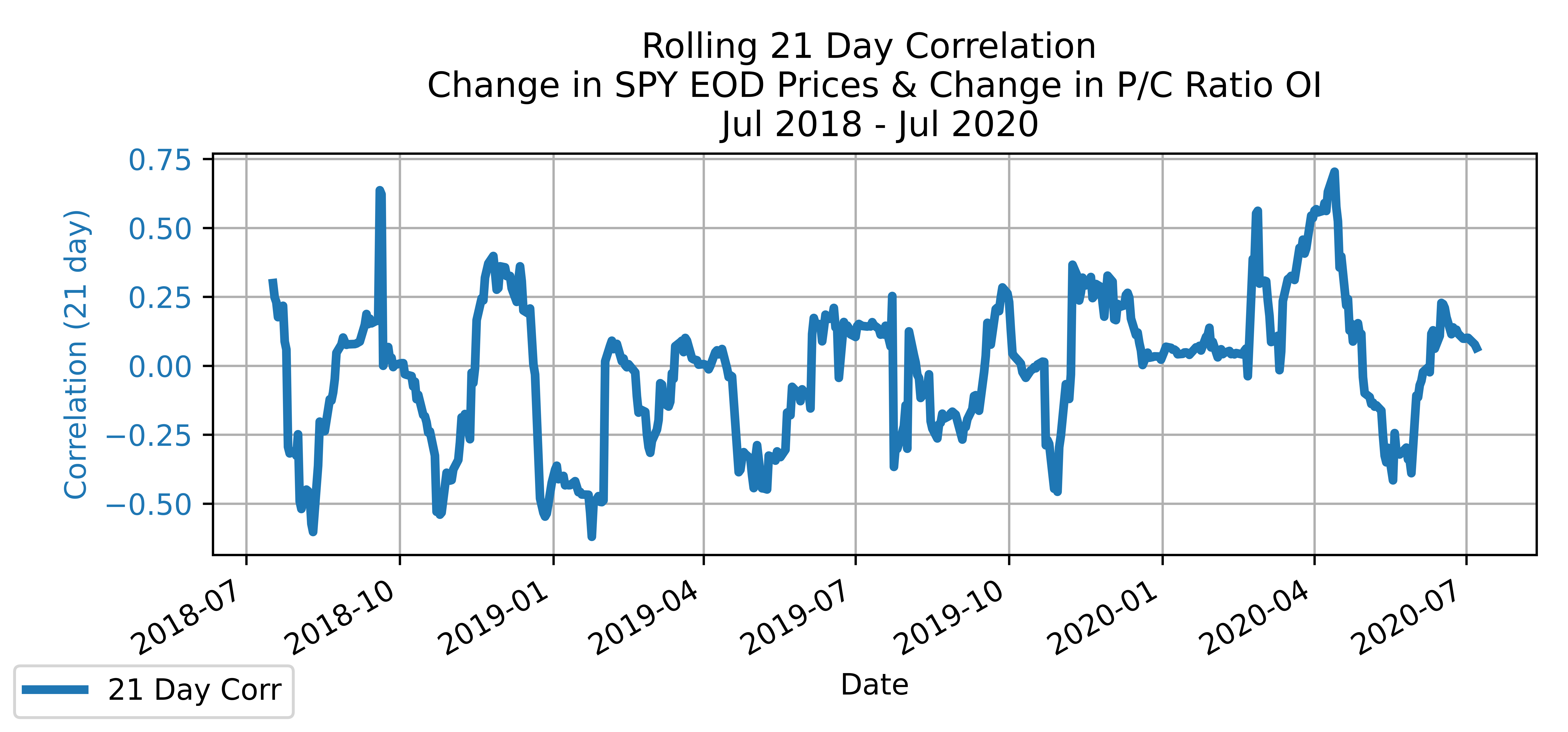One potential explanation for the oscillation is that during longer periods of upward trending prices (bull run), more Puts are purchased as protection so investors can lock in profits instead of speculating a downward turn. During times where sentiment is bearish the correlation between the change in price and change in metric is strongly negative. Leveraging this as a signal, some simple strategies were backtested using data from January 2005 through September 2021 on the ticker SPY.

Results across all the variations were relatively similar. Below are the strategy descriptions and their backtest results when using the Puts Percentage based on Open Interest variation.

Strategy Description
Buy & Hold Purchase the asset at the beginning of the period and hold until the end of the period.
P/C Ratio 95% Confidence Interval If the current ratio is more than 2 standard deviations (3-month) from it's average (3-month) then sell, if it is less than 2 standard deviations from its average then buy.
P/C Ratio 95% Confidence Interval when Correlation < -0.5 If the current ratio is more than 2 standard deviations (3-month) from it's average (3-month) and the correlation (3-month) is less than -0.5 then sell, if it is less than 2 standard deviations from its average and the correlation (3-month) is less than -0.5 then buy.
P/C Ratio Static Bounds If the P/C Ratio Percentage is greater than 0.7 then buy, less than 0.5 then sell.

*Results in table are based on the Puts Percentage using Open Interest

Strategy Buy & Hold PC Ratio C.I. PC Ratio C.I and (ρ) PC Ratio Static Bounds
Total Return 268.88% 208.33% 177.70% 168.31%
Sharpe 0.59 0.51 0.70 0.46
Sortino 1.00 0.89 4.49 0.80
Max Drawdown -56.77% -56.20% -33.92% -56.75%
Yearly Mean 9.58% 8.52% 7.02% 7.87%
Yearly Vol 16.33% 16.64% 10.04% 17.11%
Best Year 29.60% 30.37% 28.88% 29.58%
Worst Year -38.48% -38.46% -6.25% -38.46%

*Results in table are based on the Puts Percentage using Open Interest

Although there are no standout backtests, when compared to simply buying and holding, the return from the ratio and correlation signal (as previously seen above to be highly significant in the explanation of next day returns) saw a slightly worse return but better Sharpe (still less than 1) and Sortino (just over 4 which is noteworthy) ratios as drawdowns were significantly reduced over the period evidencing that this signal can provide a not so far off market return with much less downside risk.

These findings indicate that the P/C Ratio, and its many variations, can be utilized as a strong indicator/signal in any alpha model or factor model.